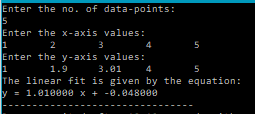# Linear Fitting – C PROGRAM

In this post I am sharing a C program that uses the least-squares approximation (also known as Chi square minimization) to find the best fit line to a series of data-points. Or in other words, the equation of a line that best fits a given set of data.

The equation of a line is given by:$y=mx+c$
where ‘m’ is the slope and ‘c’ is the intercept.

So we will need to determine these constants in the above equation.

We will be using the Least Squares Method to achieve this.

Let’s say you have n data points:$x_i$ and$y_i$ .
Then the fitted function can be calculated by minimizing:$\boxed{err=\Sigma^n_{i=1}(Y_i-(mx_i+c))^2}$
where,$Y_i$ are the fitted points, given by$Y_i=m x_i+c$ .
Minimization is done by taking partial derivatives with respect to ‘m’ and ‘c’ respectively and equating to 0.

Skipping all the math, we get the following formulae for$m$ and$c$:$\boxed{m= \frac{n\Sigma x_iy_i-\Sigma x_i \Sigma y_i}{n\Sigma x_i^2-(\Sigma x_i)^2} }$$\boxed{c= \frac{\Sigma x_i^2 \Sigma y_i -\Sigma x_i \Sigma x_iy_i}{n\Sigma x_i^2-(\Sigma x_i)^2} }$

You can refer to this link for a detailed proof.

The code is pretty much easy to understand. If you still have any doubts leave them in the comments section down below.

### CODE:

/******************************************************
****************Chi-square linear fitting**************
******************************************************/
#include<stdio.h>
#include<math.h>
/*****
Function that calculates and returns the slope of the best fit line
Parameters:
N: no. of data-points
x[N]: array containing the x-axis points
y[N]: array containing the corresponding y-axis points
*****/
double slope(int N, double x[N], double y[N]){
double m;
int i;
double sumXY=0;
double sumX=0;
double sumX2=0;
double sumY=0;
for(i=0;i<N;i++){
sumXY=sumXY+x[i]*y[i];
sumX=sumX+x[i];
sumY=sumY+y[i];
sumX2=sumX2+x[i]*x[i];
}
sumXY=sumXY/N;
sumX=sumX/N;
sumY=sumY/N;
sumX2=sumX2/N;
m=(sumXY-sumX*sumY)/(sumX2-sumX*sumX);
return m;
}
/*****
Function that calculates and returns the intercept of the best fit line
Parameters:
N: no. of data-points
x[N]: array containing the x-axis points
y[N]: array containing the corresponding y-axis points
*****/
double intercept(int N, double x[N], double y[N]){
double c;
int i;
double sumXY=0;
double sumX=0;
double sumX2=0;
double sumY=0;
for(i=0;i<N;i++){
sumXY=sumXY+x[i]*y[i];
sumX=sumX+x[i];
sumY=sumY+y[i];
sumX2=sumX2+x[i]*x[i];
}
sumXY=sumXY/N;
sumX=sumX/N;
sumY=sumY/N;
sumX2=sumX2/N;
c=(sumX2*sumY-sumXY*sumX)/(sumX2-sumX*sumX);
return c;
}
main(){
int N;
printf("Enter the no. of data-points:\n");
scanf("%d",&N);
double x[N], y[N];
printf("Enter the x-axis values:\n");
int i;
for(i=0;i<N;i++){
scanf("%lf",&x[i]);
}
printf("Enter the y-axis values:\n");
for(i=0;i<N;i++){
scanf("%lf",&y[i]);
}
printf("The linear fit is given by the equation:\n");
double m=slope(N,x,y);
double c=intercept(N,x,y);
printf("y = %lf x + %lf",m,c);
}



### OUTPUT:So that’s it.
You now have the value of ‘m'(slope) and ‘c'(intercept) and thus the linear fit:$y=mx + c$
You can refer to the following links for more info:
Linear Fitting – Lab Write-Up
Linear Fitting – C++ Program
Linear Fitting – Scilab Code
Curve Fit Tools – Android App (using the above code)
Curve Fit Tools – Documentation
Curve Fit Tools – Play Store
Curve Fit Tools – GitHub Repository
Curve Fitters – Scilab Toolbox[wpedon id="7041" align="center"]